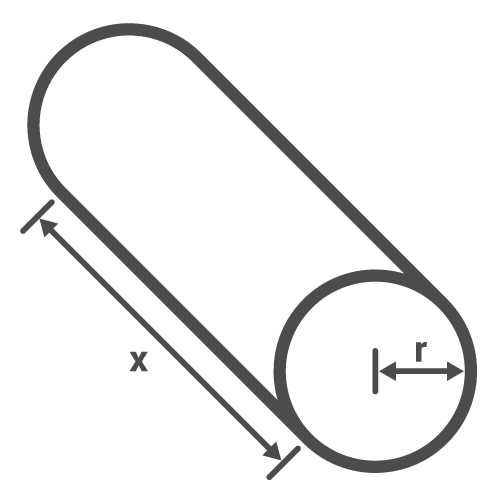# Waveguide Calculator (Circular)

## This tool calculates the cutoff frequency of a circular waveguide

(Hz)

### Overview

A waveguide is a transmission line for microwave frequency signals and commonly comes in circular or rectangular form. The waveguide essentially acts like a high pass filter and the frequency limit is known as the cutoff frequency. This calculator computes the cutoff frequency of a circular waveguide if its radius is known.### Equation

$$f_{c} = \frac{1.8412c}{2\pi r}$$

Where:

$$f_{c}$$ = circular waveguide cutoff frequency

$$c$$ = speed of light = 3 x 108 m/s

$$r$$ = radius of the waveguide

### Applications

Waveguides are hollow tubes which allows the propagation of microwave signals on a given cutoff frequency. They are easier to manufacture than coaxial cables because there is no need for a central or inner conductor. They can also handle very high powers due to the very high dielectric breakdown of air (which is what is found inside the waveguide). Radiation loss is also minimal in a waveguide compared to a coaxial cable. These are the reasons why the waveguide is the preferred transmission line in radar systems.

The coaxial cable is more advantageous compared to the waveguide when it comes to size and cost. Waveguide walls are also not perfect conductors which means heating (I2R) loss is common.